When I studied high school geometry, I noticed that many homework problems involved right triangles whose side lengths were integers. The canonical example is the 3-4-5 right triangle, which has legs of length 3 and 4 and a hypotenuse of length 5. The triple (3, 4, 5) is called a Pythagorean triple because it satisfies the Pythagorean theorem: 32 + 42 = 52. Similarly, (5, 12, 13) and (7, 24, 25) are Pythagorean triples that sometimes appear in geometry textbooks.

In general, a triple of natural numbers (a, b, c) is a Pythagorean triple if a2 + b2 = c2. Obviously if (a, b, c) is a Pythagorean triple, then so is (ka, kb, kc) for any natural number k. Geometrically, this transformation is not very interesting because it simply scales a right triangle into a similar triangle that has the same shape. I did not learn any trick in high school that enabled me to start with a Pythagorean triple and generate a new, geometrically different, right triangle.

### Generating Pythagorean triples

Because my high school geometry book reused the same three or four triples over and over again, I assumed that it is difficult to generate Pythagorean triples. In fact, that is not the case: There are several formulas for generating Pythagorean triples, some of which were known to the ancient Greeks.

One interesting algorithm comes from the 20th-century and involves linear transformations via matrices. Here's how it works. Write any Pythagorean triple, v, as a row vector. Then the linear transformation v*L is a new Pythagorean triple, where L is any of the three following linear transformations: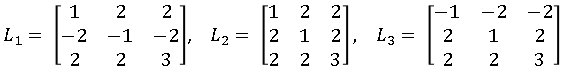For example, if you represent v = (3, 4, 5) as a row vector, then v*L1 = (5, 12, 13). You can multiply on the right again to get another new triple, such as v*L1*L3 = (45, 28, 53).

In fact, more is true. A primitive Pythagorean triple is a triple such that the three numbers have no nontrivial divisors, that is, they are relatively prime. It turns out that all primitive Pythagorean triples can be obtained by iteratively applying one of three linear transformations to the triple (3, 4, 5). In other words, the triple (3, 4, 5) is the "parent" of all primitive Pythagorean triples!

That is a fabulous result. It means that you can write a program that uses matrix multiplication to produce arbitrarily many primitive Pythagorean triples, as follows:

• Apply L1, L2, and L3 to create the first generation of children.
• Apply the three transformations to each of the children to create a generation of nine grandchildren.
• Continue this process. The ith generation will contain 3i triples.

You can implement this algorithm in the SAS/IML matrix language as follows:

```proc iml; start GenerateTriples(depth=3); L1 = { 1 2 2, -2 -1 -2, 2 2 3}; L2 = { 1 2 2, 2 1 2, 2 2 3}; L3 = {-1 -2 -2, 2 1 2, 2 2 3}; NumTriples = sum(3##(0:Depth)); /* total number of triples */ triples = j(NumTriples,3,.); /* allocate array for results */ triples[1,] = {3 4 5}; /* parent triple */ k = 1; n = 2; do i = 1 to Depth; /* for each generation */ do j = 1 to 3##(i-1); /* generate 3##i children */ x = triples[k,]; triples[n,] = x*L1; triples[n+1,] = x*L2; triples[n+2,] = x*L3; k = k + 1; n = n + 3; end; end; return( triples ); finish;   p = GenerateTriples(); print p;```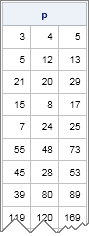The parent and the first three generations account for 1 + 3 + 9 + 27 = 40 triples. The list contains some old familiar friends, as well as a few strangers that I haven't met before.

### The distribution of Pythagorean triples

What do the Pythagorean triples look like if you plot their location in a Cartesian coordinate system? It turns out that they make fascinating patterns!

Because the hypotenuse length is a function of the leg lengths, it suffices to plot the locations of a and b in the coordinate plane. However, it turns out that when the algorithm produces a particular triple such as (5, 12, 13), it does not produce the symmetric triple (12, 5, 13). To make the pattern more symmetric, we can to manually add the triple (b, a, c) whenever the triple (a, b, c) appears.

The following SAS/IML statements construct many primitive Pythagorean triples and create a scatter plot of the ones for which a and b are both less than 4,500:

```p = GenerateTriples(15); /* generate many triples */ m = p[ loc(p[,1]<=4500 & p[,2]<=4500), ]; /* exclude large ones */ mm = m // m[,{2 1 3}]; /* generate symmetric pairs */   ods graphics / width=600px height=600px; title "Primitive Pythagorean Triples"; title2 "Depth = 15, Symmetric"; call scatter(mm[,1], mm[,2]) procopt="aspect=1" option="markerattrs=(size=3 symbol=SquareFilled)";```

The image is shown at the beginning of this article. You can see certain parabolic curves that have a high density of points. There is a similar plot in Wikipedia that includes nonprimitive triples. The nonprimitive triples "fill in" some of the apparent "gaps" in the pattern.

These interesting patterns hint at deep relationships in number theory between Diophantine equations and the geometry of the solutions. Are you tempted to generate a similar image for the generalized equation a3 + b3 = c3? Don't bother: Fermat's Last Theorem states that this equation has no integer solutions!

tags: Just for Fun, MathWhen I studied high school geometry, I noticed that many homework problems involved right triangles whose side lengths were integers. The canonical example is the 3-4-5 right triangle, which has legs of length 3 and 4 and a hypotenuse of length 5. The triple (3, 4, 5) is called a Pythagorean triple because it satisfies the Pythagorean theorem: 32 + 42 = 52. Similarly, (5, 12, 13) and (7, 24, 25) are Pythagorean triples that sometimes appear in geometry textbooks.

In general, a triple of natural numbers (a, b, c) is a Pythagorean triple if a2 + b2 = c2. Obviously if (a, b, c) is a Pythagorean triple, then so is (ka, kb, kc) for any natural number k. Geometrically, this transformation is not very interesting because it simply scales a right triangle into a similar triangle that has the same shape. I did not learn any trick in high school that enabled me to start with a Pythagorean triple and generate a new, geometrically different, right triangle.

### Generating Pythagorean triples

Because my high school geometry book reused the same three or four triples over and over again, I assumed that it is difficult to generate Pythagorean triples. In fact, that is not the case: There are several formulas for generating Pythagorean triples, some of which were known to the ancient Greeks.

One interesting algorithm comes from the 20th-century and involves linear transformations via matrices. Here's how it works. Write any Pythagorean triple, v, as a row vector. Then the linear transformation v*L is a new Pythagorean triple, where L is any of the three following linear transformations:For example, if you represent v = (3, 4, 5) as a row vector, then v*L1 = (5, 12, 13). You can multiply on the right again to get another new triple, such as v*L1*L3 = (45, 28, 53).

In fact, more is true. A primitive Pythagorean triple is a triple such that the three numbers have no nontrivial divisors, that is, they are relatively prime. It turns out that all primitive Pythagorean triples can be obtained by iteratively applying one of three linear transformations to the triple (3, 4, 5). In other words, the triple (3, 4, 5) is the "parent" of all primitive Pythagorean triples!

That is a fabulous result. It means that you can write a program that uses matrix multiplication to produce arbitrarily many primitive Pythagorean triples, as follows:

• Apply L1, L2, and L3 to create the first generation of children.
• Apply the three transformations to each of the children to create a generation of nine grandchildren.
• Continue this process. The ith generation will contain 3i triples.

You can implement this algorithm in the SAS/IML matrix language as follows:

```proc iml; start GenerateTriples(depth=3); L1 = { 1 2 2, -2 -1 -2, 2 2 3}; L2 = { 1 2 2, 2 1 2, 2 2 3}; L3 = {-1 -2 -2, 2 1 2, 2 2 3}; NumTriples = sum(3##(0:Depth)); /* total number of triples */ triples = j(NumTriples,3,.); /* allocate array for results */ triples[1,] = {3 4 5}; /* parent triple */ k = 1; n = 2; do i = 1 to Depth; /* for each generation */ do j = 1 to 3##(i-1); /* generate 3##i children */ x = triples[k,]; triples[n,] = x*L1; triples[n+1,] = x*L2; triples[n+2,] = x*L3; k = k + 1; n = n + 3; end; end; return( triples ); finish;   p = GenerateTriples(); print p;```The parent and the first three generations account for 1 + 3 + 9 + 27 = 40 triples. The list contains some old familiar friends, as well as a few strangers that I haven't met before.

### The distribution of Pythagorean triples

What do the Pythagorean triples look like if you plot their location in a Cartesian coordinate system? It turns out that they make fascinating patterns!

Because the hypotenuse length is a function of the leg lengths, it suffices to plot the locations of a and b in the coordinate plane. However, it turns out that when the algorithm produces a particular triple such as (5, 12, 13), it does not produce the symmetric triple (12, 5, 13). To make the pattern more symmetric, we can to manually add the triple (b, a, c) whenever the triple (a, b, c) appears.

The following SAS/IML statements construct many primitive Pythagorean triples and create a scatter plot of the ones for which a and b are both less than 4,500:

```p = GenerateTriples(15); /* generate many triples */ m = p[ loc(p[,1]<=4500 & p[,2]<=4500), ]; /* exclude large ones */ mm = m // m[,{2 1 3}]; /* generate symmetric pairs */   ods graphics / width=600px height=600px; title "Primitive Pythagorean Triples"; title2 "Depth = 15, Symmetric"; call scatter(mm[,1], mm[,2]) procopt="aspect=1" option="markerattrs=(size=3 symbol=SquareFilled)";```

The image is shown at the beginning of this article. You can see certain parabolic curves that have a high density of points. There is a similar plot in Wikipedia that includes nonprimitive triples. The nonprimitive triples "fill in" some of the apparent "gaps" in the pattern.

These interesting patterns hint at deep relationships in number theory between Diophantine equations and the geometry of the solutions. Are you tempted to generate a similar image for the generalized equation a3 + b3 = c3? Don't bother: Fermat's Last Theorem states that this equation has no integer solutions!

tags: Just for Fun, MathMany people enjoy solving word games such as the daily Cryptoquote puzzle, which uses a simple substitution cipher to disguise a witty or wise quote by a famous person. A common way to attack the puzzle is frequency analysis. In frequency analysis you identify letters and pairs of letters (bigrams) that occur often in the enciphered text. It is a fact that certain letters (e, t, a, o, i, n,....) and bigrams (th, he, in, er, an, re, on,...) appear frequently in English text. You can use this fact to guess that the most frequently occurring symbol in the text might represent e, t, or a. You can use the bigram frequencies in the text to help you make statistically likely guesses.

Usually frequency analysis is used for the initial guesses. Soon recognizable snippets of words begin to appear in the partially deciphered text, and you can often guess small words such as 'the', 'and', 'of', and 'for'. Then, like pulling a string on a sweater, the puzzle unravels. Use the context of the message to guess the remaining letters.

This article shows how to use frequency analysis to solve a cryptogram. I use a computer program to count the frequency of letters and bigrams and to quickly substitute guessed letters into the puzzle. However, the computer program merely provides an implementation of a tedious and repetitive task. You can perform the same analysis with pencil and paper. The program does not make any guesses itself, but merely makes it easier for a human to make a guess. (Type 'cryptogram solver' into an internet seach engine if you want an online solver.)

I use the convention that capital letters are symbols for the enciphered text whereas lowercase letters indicate the plaintext. Thus the plaintext message "word games are fun" might be enciphered as "RHES ADLUI DEU VYP." A partially deciphered solution might appear as "RHrS AaLeI are VYn," where the uppercase letters represent yet-to-be-solved letters.

### Some functions that help decipher Cryptoquotes

• The PrintFreqRef routine prints reference tables that show how often certain letters and pairs of letters appear in English text. The tables are the results from previous blog posts and show the most frequently appearing letters, the most frequent bigrams, and the most frequent double-letter bigrams.
• The FreqAnalysis routine reports the distribution of letters, bigrams, and double letters in the enciphered quote.
• The SplitByWords function constructs an array from a quote. Each word is on one row; each letter is in a separate column. The main purpose of this routine is so that I can say "look at the 7th word" and you will be able to find it easily.
• The ApplySubs function enables you to quickly see the result of replacing one or more cipher symbols with a guess. You can use the function to replace 'Q' with 't' and 'F' with 'e' and see whether the substitutions appear to make sense in the quote. If the substitution results in nonsense—such as 'te' as a two-letter word—then the substitution was not good and you can try a different guess.

### Solving the Cryptoquote by using frequency analysis

The following statements start PROC IML, load the utility modules, and print the reference tables that show the expected frequencies of letters and bigrams in English text.

```proc iml; load module=_all_; /* load the utility modules */ run PrintFreqRef();```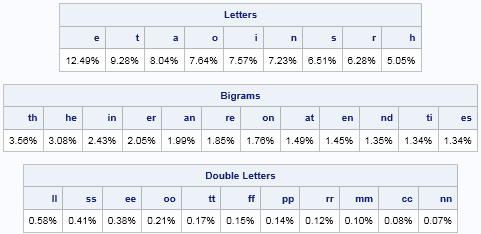The reference tables show the relative frequencies of the most common letters, such as e, t, a, o, i, n, and s. The most frequent pairs of letters are th, he, in, er, and so forth. The most common double-letter bigrams are ll, ss, ee, oo, and so forth. If you like to solve word puzzles by pencil and paper, you can print this table and keep a copy in your wallet or purse. Click the table to get it to appear on a separate web page.

Now let's look at an enciphered quote and perform a frequency analysis on its symbols:

```msg = "KBSCS OCS KPUSH XBSW DOCSWKBTTG HSSUH WTKBPWJ " + "IAK LSSGPWJ KBS UTAKB KBOK IPKSH MTA. " + "~ DSKSC GS FCPSH"; run FreqAnalysis(msg);```These tables look similar to the reference tables, but these tables are specific to this quote. You can create these table by hand if you have the time and patience. In this quote, almost 20% of the symbols are an S. The next most frequent symbols are K and B. Furthermore, the bigrams KB and BS appear frequently. This co-occurrence of three symbols and two bigrams is a "trifecta" that gives strong statistical evidence that S=e, K=t, and B=h. The S=e guess is further supported by the fact that SS appears twice in the message, and 'ee' is a common double-letter bigram.

Let's make the substitutions:

```w = SplitByWords(msg); /* split into array */ rownum = char(1:nrow(w)); /* row numbers, for reference */ w1 = ApplySubs(w, {K B S}, {t h e}); /* guess K=t, B=h, and S=e */ print w1[r=rownum];```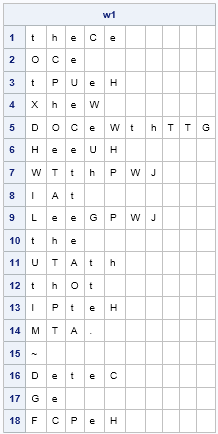It looks like we made a great guess! The first word is probably 'there', so the next substitution should include C=r. This guess is bolstered by the fact that CS appears several times in this message, and 're' is a frequent bigram. (The first word could also be 'these', but 'se' occurs less often than 're'.)

If that guess is correct, then we can further guess that O=a because that makes the second word 'are' and the 12th word 'that'.

To generate another guess, notice that the double-letter bigram TT appears in this quote in the 5th word. The bigram follows the plaintext bigram 'th', so TT must represent a double vowel. Because we have already deduced the symbol for 'e', we guess that TT is 'oo', which is the only other common double-vowel bigram. Therefore T=o.

Lastly, the 16th word is the author's first name. Since C=r, we guess that the first name might be 'peter'. The following statement makes these substitutions:

```w2 = ApplySubs(w1, {O C}, {a r}); w3 = ApplySubs(w2, {T D}, {o p}); print w3[r=rownum];```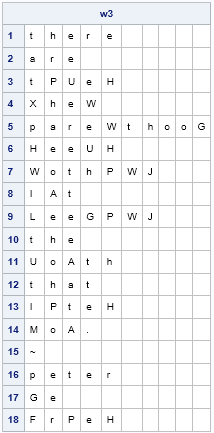Frequency analysis can suggest additional guesses. The symbols H, P, and W appear frequently in the enciphered quote. We also see that SH (=eH) is a frequent bigram. From the bigram frequency analysis, we might guess that H=n or H=s. By looking at the partially deciphered quote, it looks like W=n because the 5th word is probably 'parenthood', so we will guess H=s.

By looking at where the symbol P appears in the quote, you might guess that P is a vowel. The remaining vowel that occurs frequently is 'i'. This guess makes sense because the trigram PWJ appears twice, and this trigram could be 'ing'.

The following statement make these substitutions.

```w4 = ApplySubs(w3, {H W G P J}, {s n d i g}); print w4[r=rownum];```

Click this link to see the partially deciphered text that results from these substitutions. At this point, frequency analysis has revealed all but seven of the symbols in the enciphered quote. The remaining symbols are easily deduced by looking at the partially deciphered words and guessing the remaining letters. The deciphered quote is:

There are times when parenthood seems nothing but feeding the mouth that bites you.
~ Peter de Vries

Frequency analysis enabled us to guess values for certain frequently occurring symbols. By correctly guessing these key symbols, the puzzle was solved. Notice that the frequency of bigrams was essential in this process. If you use only the frequency of single letters, you might be able to guess the 'e' and 't', but the other letters are indistinguishable by looking at the empirical frequencies. However, by using bigrams, you can guess more symbols. For example, in this puzzle the bigrams helped us to discover the symbols for 'h', 'o', 's', and 'n'.

Of course, this process is not always linear. Sometimes you will make a guess that turns out to be wrong. In that case, eliminate the wrong guess and try another potential substitution.

To conclude this article, I will leave you with another Cryptoquote. I will generate the frequency analysis for you, in case you want to solve the puzzle by hand. You can download the SAS/IML program that deciphers this quote. The program contains comments that explain how frequency analysis is used to solve the puzzle.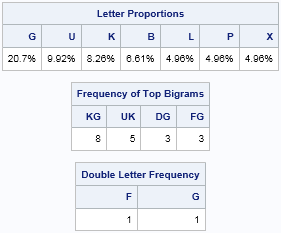CG EKLVFX WLU MVXZG IGLIFG YH UKGSB IGPQ LR GNDGFFGWDG; YVU YH UKG XSEUPWDG UKGH KPTG UBPTGFGX RBLA UKG ILSWU CKGBG UKGH EUPBUGX.
~ KGWBH CPBX YGGDKGB
tags: Ciphers, Just for FunMy previous blog post describes how to implement Conway's Game of Life by using the dynamically linked graphics in SAS/IML Studio. But the Game of Life is not the only kind of cellular automata. This article describes a system of cellular automata that is known as Wolfram's Rule 30. In this blog post I show how to compute and visualize this system in SAS by using traditional PROC IML and a simple heat map.

### Wolfram's Rule 30

Conway's Game of Life is a set of rules for evolving cellular automata on a two-dimensional grid. However, one-dimensional automata are simpler to describe and to compute. A well-known one-dimensional example is Wolfram's Rule 30 (1983, Rev. Mod. Phys.). Consider a sequence of binary symbols, such as 0 and 1. An initial configuration determines the next generation by using the following two rules for each cell:

1. If the cell and its right-hand neighbor are both 0, then the new value of the cell is the value of its left-hand neighbor.
2. Otherwise, the new value of the cell is the opposite of the value of its left-hand neighbor.

The rules are often summarized by using three-term sequences that specify the value of the left, center, and right cells. The sequence 100 means that the center cell is 0, its left neighbor is 1, and its right neighbor is 0. According to the first rule, the value of the center cell for the next generation will be 1. The sequence 000 means that the center cell and both its neighbors are 0. According to the first rule, the value of the center cell for the next generation will be 0. All other sequences are governed by the second rule. For example, the sequence 010 implies that the center cell for the next generation will be 1, which is the opposite of the value of the left neighbor.

There are several ways that you can implement Rule 30 in a matrix language such as SAS/IML. The following SAS/IML program defines a function that returns the next generation of binary values when given the values for the current generation. As for the Game of Life, I define the leftmost and rightmost cells to be neighbors. The evolution function is vectorized, which means that no loops are used to compute a new generation from the previous generation. The second function computes each new generation as a row in a matrix. You can print that matrix or use the HEATDISC subroutine in SAS/IML 13.1 to create a black-and-white heat map of the result.

```proc iml; start Rule30Evolve(x); /* x is binary row vector */ y = x[ncol(X)] || x || x; /* extend x periodically */ N = ncol(y); j = 2:N-1; xj = y[,j]; /* the original cells */ R = y[,j+1]; L = y[,j-1]; /* R=right neighbor; L=left neighbor */ idxSame = loc(xj=0 & R=0); /* x00 --> center cell is x */ if ^IsEmpty(idxSame) then y[,idxSame+1] = L[,idxSame]; idxDiff = setdif(1:N-2, idxSame); /* otherwise, center cell is ^x */ if ^IsEmpty(idxDiff) then y[,idxDiff+1] = ^L[,idxDiff]; return( y[,j] ); finish;   start Rule30(x0, Iters=ceil(ncol(x0)/2)); N = ncol(x0); M = j(Iters,N,0); /* initialize array to 0 */ M[1,] = x0; /* set initial configuration */ do i = 2 to Iters; M[i,] = Rule30Evolve(M[i-1,]); /* put i_th generation into i_th row */ end; return( M ); finish;   N = 101; x0 = j(1,N,0); /* initialize array to 0 */ x0[ceil(N/2)] = 1; /* set initial condition for 1st row */ M = Rule30(x0, N); ods graphics / width=800px height=600px; call heatmapdisc(M) displayoutlines=0 colorramp={White Black} title = "Wolfram's Rule 30 (N=101)";```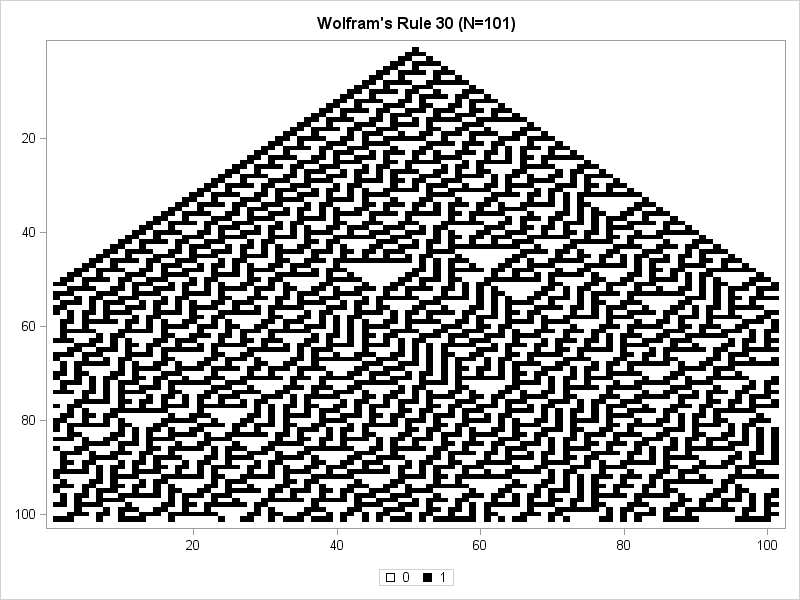The discrete heat map uses white to indicate cells that are 0 and black to indicate cells that are 1. The initial configuration has 100 cells of white and a single black cell in the middle of the array. That configuration is shown in the first row of the heat map. (Click to enlarge.) The second generation has three black cells in the middle, as shown in the second row. The central region of cells grows two cells larger for each generation. For the first 50 generations, the left boundary is always 001 and the right boundary alternates between 111 and 001. The pattern of the interior cells is not periodic, although certain interesting patterns appear, such as "martini glasses" of various sizes.

After 50 generations, the left and right edges begin to interact in a nontrivial way. Periodicity at the edges is replaced by irregular patterns.

Supposedly the evolution of the middle cell of the array generates nearly random 0-1 values. Mathematica, a computer software package created by Stephen Wolfram, uses the central column of a Rule-30 cellular automata as a random number generator. From a simple deterministic rule comes complex behavior that seems indistinguishable from randomness.

The heat map shows all generations at a single glance by stacking them as rows in a single image. This is different from the Game of Life visualization, which used animation to show how an initial configuration evolves from one generation to the next. You can also use animation to visualize the Rule-30 system. The following animated GIF shows the same 100 generations of an initial configuration that consists of a single black cell in the middle of a 101-cell array. This animation shows how that configuration evolves in time.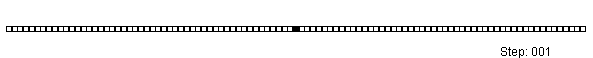It is fun to play with Rule 30. To begin, I suggest an array of 21 cells that evolves for 20 generations. The resulting black-and-white heat map is small enough to study, and you can use the DISPLAYOUTLINES=1 option to better visualize which cells are white during the evolution. You can invent your own initial configuration or use random initial conditions, as shown below:

```N = 21; x0 = T( randfun(N, "Bernoulli", 4/N) ); /* random; expect 4 black cells */ M = Rule30(x0); call heatmapdisc(M) displayoutlines=1 colorramp={White Black} title = "Wolfram's Rule 30 (N=21)";```

Enjoy!A colleague jokingly teases me whenever I write a blog that demonstrates how to write fun and exciting programs by using SAS software. "Why do you get to have all the fun?" he mock-chides.

### Cellular automata and Conway's Game of Life

Conway's Game of Life is a set of simple rules that give rise to beautiful regular and irregular patterns. The rules describes how cellular automata live or die based on the presence or absence of neighbors on a rectangular grid. This dynamical system is a "game" because it is fun to create some initial distribution of automata and watch the evolution of the system from that initial condition. Like many programmers, I wrap the edges of the grid so that the cells on the right and left sides of the grid are neighbors, and similarly for the cells on the top and bottom edges.

The following rules were used to evolve the automata:

• Birth Rule: An organism is born into any empty cell that has exactly three living neighbors.
• Survival Rule: An organism with either two or three neighbors survives from one generation to the next.
• Death Rule: An organism with four or more neighbors dies from overcrowding. An organism with fewer than two neighbors dies from loneliness.

### The Game of Life in SAS/IML Studio

Almost every beginning programmer has attempted to program the Game of Life. In a matrix language such as SAS/IML, the implementation of the rules are particularly easy and compact. I first implemented the Game of Life in SAS/IML Studio in 2001. My program is part of the standard set of demo programs that are distributed with SAS/IML Studio. To access the program from the SAS/IML Studio menu, select File ⇒ Open ⇒ File. Then click Go to Installation directory. Select the Programs and Demos folders, then open the file Life.sx.

When you run the program, you are prompted for the size of a square grid (I like 20 rows and columns) and told to click on the grid to create an initial distribution of the automata. (Hold down the SHIFT key while you click.) The following animated GIF shows 100 steps that evolve from a simple initial condition that contains an "exploder" pattern and a "glider" pattern.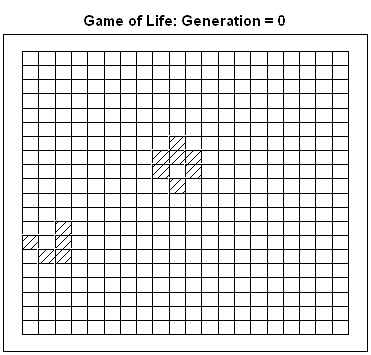The interesting thing about this implementation in SAS/IML Studio is that I didn't program the animation at all. The "live" automata are observations that are selected in the grid. The program figures out which cells are alive and dead at the next generation and then simply tells the in-memory copy of the data to update the selected observations. The plot automatically updates to reflect the newly selected observations. To learn more about dynamically linked graphics and how to select observations in SAS/IML Studio, see my book Statistical Programming with SAS/IML Software, especially Chapters 6 and 10.

Recall that SAS/IML Studio is a free download and can be installed on a Windows PC and used by anyone who has a license for SAS/IML and SAS/STAT software. Unfortunately, SAS/IML Studio is not available for users of the free SAS University Edition. If you do not have access to SAS/IML Studio, here is a version of the Life.sx program. You can use the computational portions of the program in PROC IML, even though you cannot create the IMLPlus graphics.

### Creating an animated GIF in SAS/IML Studio

When you run the program in SAS/IML Studio, the graphics are displayed on your PC monitor. So how did I create an animated GIF to embed in this blog post? It was surprisingly easy! In the IMLPlus program, the on-screen graph is controlled through a Java variable called plot. You can use the SaveToFile method to create a bitmap version of the graph. If you call that method within the loop that updates the graph for each new generation of automata, you get a sequence of bitmaps in some local directory, like this:

```path = 'C:TempAnimGifLife'; /* directory to store images */ plot.SaveToFile(path+"Life000.bmp"); /* save the initial configuration */ do i = 1 to nGenerations; /* for each new generation */ run update_generation( grid ); /* compute new configuration for automata */ run Grid2Selection( dobj, grid ); /* update the selected observations */ plot.SetTitleText("Game of Life: Generation = "+char(i,3)); /* update title */ suffix = trim(putn(i, "Z3.")); /* 001, 002, ..., 100 */ plot.SaveToFile(path+"Life"+suffix+".bmp"); /* save image to Life001.bmp */ end;```

After creating 100 bitmap images in a directory, you can create an animated GIF by using a program that stitches the images together in sequence. I used a freeware Java program called GiftedMotion, which does not require you to install any software. Just download the JAR file to your desktop, double click, and navigate to the directory that contains the images.Last week Chris Hemedinger posted an article about spam that is sent to SAS blogs and discussed how anti-spam software helps to block spam. No algorithm can be 100% accurate at distinguishing spam from valid comments because of the inherent trade-off between specificity and sensitivity in any statistical test. Therefore, some spam comments slip through the anti-spam filter, and I get the pleasure of reading the comments and deciding whether to allow them to appear on my blog, or whether to manually mark them as spam.

### Why spammers submit comments to blogs

When I first started getting spam comments, I wondered what the spammers were trying to achieve. A typical spam comment seems fairly innocuous. Here are some actual spam comments that I have received:

• Thanks for a marvelous posting! I certainly enjoyed reading it, you can be a great author.
• Thank you for the good writeup. It in fact was a amusement account it.
• If all bloggers made good content as you did, the internet will be much more useful than ever before.

Yes, some of the grammar and word choices are strange, but these comments are not much different from some legitimate comments that I have received from legitimate readers whose native language is not English. What makes me sure that these are spam comments?

Along with each of these comments, the commenter included a URL link to some web site. The URL in a typical spam comment links to a web site that advertises cheap "name brand" merchandise, Russian brides, or get-rich-quick schemes. The spammers get paid for each link that they can successfully embed somewhere on the web, such as on my blog. As you might know, internet search engines use the number of "incoming links" as a measure of how important a web site is, and therefore how high it should appear in the search results. The goal of the blog spammer is to embed many links in many blog articles so that internet search engines rank their sponsoring web site highly when someone searches for something like "cheap viagra."

The link is not always embedded in the comment itself. When you comment on a blog, you have the option to include your name and to link to your personal web site. In a legitimate comment, the links points to the commenter's blog or business; spammers link to their sponsoring URL.

### How spammers create random comments

As you can see from the sample comments, spammers try to construct complimentary but fairly generic message that they can submit regardless of an article's content.

Suppose that a spammer decides to construct the following generic message: Your blog is truly wonderful. He could write a program that submits this comment to a million blogs. However, he would not be very successful because anti-spam software can block this simple attack by applying simple logic: IF the comment is 'Your blog is truly wonderful' AND the URL field is filled in, THEN classify the comment as spam.

To attempt to defeat anti-spam software, spammers randomly generate comments by using synonyms for the nouns, verbs, and adjectives that appear in the comment. For example, synonyms for "blog" include "post" and "article." A synonym for "wonderful" is "marvelous," and so forth. Thus the spammer could modify his spam program to generate random messages according to the following grammatical template: Your NOUN is ADJECTIVE SUBJECT_COMPLEMENT. The result is like those Mad Libs® stories you and your sibling used to create on long car rides. You can create a huge number of possible sentences, but most of them sound silly.

It is easy to write a SAS DATA step program that generates comments that fit the grammatical template. The following program uses the RAND function to randomly generate elements of a character array and uses the CATX function to concatenate the elements into a sentence:

```data SpamComments(keep=msg); array noun{4} \$12 _temporary_ /* nouns */ ("blog","post","article","commentary"); array adj{7} \$12 _temporary_ /* adjectives */ (" ","simply","actually","truly","sincerely","honestly","really"); array sc{6} \$12 _temporary_ /* subject complements */ ("wonderful","marvelous","fastidious","judicious","superb","fantastic"); sp = " "; /* delimiter between words */ call streaminit(12345); length msg \$ 120; do i = 1 to 20; /* generate 20 messages */ noun_i = ceil(dim(noun)*rand("uniform")); /* random index into noun array */ adj_i = ceil(dim(adj)*rand("uniform")); sc_i = ceil(dim(sc)*rand("uniform")); msg = catx(sp, "Your", noun[noun_i], "is", adj[adj_i], sc[sc_i]); output; end; run;   proc print; run;```As shown by the output on the left, these randomly generated comments can be amusing. It is often obvious when spammers use a thesaurus to automatically generate dozens of synonyms for each word in their message template. Words have subtle connotations; they cannot be mixed and matched like a verbal closet of Garanimals®. I call comments like this "grammaticals."

Do you have any experience dealing with spammers? Share your experience by leaving a comment. I'm sure it will be fastidious. If all readers make astonishing comments as you do, the web will be much more useful than ever before.Yesterday I blogged about the Hilbert matrix. The (i,j)th element of the Hilbert matrix has the value 1 / (i+j-1), which is the reciprocal of an integer.

However, the printed Hilbert matrix did not look exactly like the formula because the elements print as finite-precision decimals. For example, the last column of the matrix of size 5 is {0.2, 0.1666667, 0.1428571, 0.125, 0.1111111}. A colleague jokingly asked, "shouldn't the matrix contain fractions like 1/5, 1/6, 1/7, 1/8, and 1/9?"

To his surprise, I responded that SAS can actually print the matrix elements as fractions! SAS contains the FRACTw. format, which makes it easy to print decimals as their fractional equivalent in reduced form. Here is yesterday's matrix, printed as fractions:

`print H[format=FRACT.];`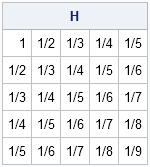I sometimes marvel at the variety of formats that are available in SAS software. From printing integers as Roman numerals to printing decimals as fractions, it seems like SAS has a format for all occasions.

tags: Just for FunMany geeky mathematical people celebrate "pi day" on March 14, because the date is written 3/14 in the US, which is evocative of the decimal representation of π = 3.14....

Most people are familiar with the decimal representation of π. The media occasionally reports on a new computational tour-de-force that approximates π to unimaginable precision. The decimal value of π has been computed to more than 10 trillion digits!

Using the decimal approximation of π is ubiquitous in mathematics, but in elementary school I learned another approximation: π ≈ 22/7. As I got older, I discovered that there were other rational approximations of π, such as 355/113.

### Continued fractions

To celebrate π day, I am going to use SAS to estimate π by using its simple continued fraction representation. Every real number x can be represented as a continued fraction: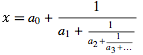In this continued fraction, the ai are positive integers. Because all of the fractions have 1 in the numerator, the continued fraction can be compactly represented by specifying only the integers: x = [a0; a1, a2, a3, ...]. Every rational number is represented by a finite sequence; every irrational number requires an infinite sequence. Notice that the decimal representation of a number does NOT have this nice property: 1/3 is rational but its decimal representation is infinite.

### The continued fraction expansion of pi

The continued fraction expansion of π is known to more than 15 billion values. The first fifteen digits are
π ≈ [3; 7, 15, 1, 292, 1, 1, 1, 2, 1, 3, 1, 14, 2, 1]
What does this mean? Well, the sequence [3; 7] corresponds to the fraction 3 + 1/7 = 22/7. Similarly, the sequence [3; 7, 15, 1] corresponds to the fraction 3 + 1/(7 + 1/(15 + 1/1)) = 355/113.

Trying to compute these fractions by hand is tedious. Why not write a SAS program to evaluate the continued fraction sequence? As the examples show, the computation starts at the end of the sequence. The computation begins by taking the reciprocal of the last number in the sequence. That values is added to the second-to-last number, and the sum is inverted. This process continues until you reach the first value. You can do this computation in the SAS DATA step (post your code in the comments!), but I have written a SAS/IML function to evaluate an arbitrary continued fraction representation. Notice that in SAS you can call the CONSTANT function to obtain a numerical approximation of π and other mathematical constants, which is useful for checking the results:

```proc iml; start ContinuedFraction(f); s = f[nrow(f)]; /* last value */ do i = nrow(f)-1 to 1 by -1; /* traverse sequence in reverse order */ s = f[i] + 1/s; end; return( s ); finish;   /* test the function by using the continued fraction expansion for pi */ f = {3, 7, 15, 1, 292, 1, 1, 1, 2, 1, 3, 1, 14, 2, 1}; s = ContinuedFraction(f); pi = constant("pi"); /* call the CONSTANT function to approximate pi */ print s[format=16.14], pi[format=16.14];```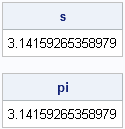As you can see, the first fifteen terms of the continued fraction expansion of π are sufficient to reproduce the first 15 digits of the decimal representation.

### Other continued fractions

Here are a few continued fraction expansions for some other irrational numbers:

• The continued fraction expansion for e ≈ 2.718281828459 shows a fascinating regular pattern: two 1s followed by an even number.
e = [2; 1, 2, 1, 1, 4, 1, 1, 6, 1, 1, 8, 1, 1, 10,....]
• The continued fraction expansion for the square root of 2 ≈ 1.414213562373095 contains a repeating sequence of 2s:
sqrt2 = [1; 2, 2, 2, 2, ...]
• The continued fraction expansion for the golden ratio φ ≈ 1.61803398874989 is a repeating sequence of 1s:
phi = [1; 1, 1, 1, 1, 1, ...]
You can use these sequences to estimate the corresponding decimal representation:
```e = ContinuedFraction({2, 1, 2, 1, 1, 4, 1, 1, 6, 1, 1, 8, 1, 1, 10}); sqrt2 = ContinuedFraction({1, 2, 2, 2, 2, 2, 2, 2, 2, 2, 2, 2, 2, 2, 2}); phi = ContinuedFraction({1, 1, 1, 1, 1, 1, 1, 1, 1, 1, 1, 1, 1, 1, 1}); print e[format=16.14], sqrt2[format=16.14], phi[format=16.14];```

The results contain less than 15-digit decimal precision. In order to obtain more precision, you would need to use more terms in the continued fraction representation.

By the way, next year's Pi Day should be special: celebrate on 3/14/15 at 9:26:53 for maximal enjoyment!Although I currently work as a statistician, my original training was in mathematics. In many mathematical fields there is a result that is so profound that it earns the name "The Fundamental Theorem of [Topic Area]." A fundamental theorem is a deep (often surprising) result that connects two or more seemingly unrelated mathematical ideas.

It is interesting that statistical textbooks do not usually highlight a "fundamental theorem of statistics." In this article I briefly and informally discuss some of my favorite fundamental theorems in mathematics and cast my vote for the fundamental theorem of statistics.

### The fundamental theorem of arithmetic

The fundamental theorem of arithmetic connects the natural numbers with primes. The theorem states that every integer greater than one can be represented uniquely as a product of primes.

This theorem connects something ordinary and common (the natural numbers) with something rare and unusual (primes). It is trivial to enumerate the natural numbers, but each natural number is "built" from prime numbers, which defy enumeration. The natural numbers are regularly spaced, but the gap between consecutive prime numbers is extremely variable. If p is a prime number, sometimes p+2 is also prime (the so-called twin primes), but sometimes there is a huge gap before the next prime.

### The fundamental theorem of algebra

The fundamental theorem of algebra connects polynomials with their roots (or zeros). Along the way it informs us that the real numbers are not sufficient for solving algebraic equation, a fact known to every child who has pondered the solution to the equation x2 = –1. The fundamental theorem of algebra tells us that we need complex numbers to be able to find all roots. The theorem states that every nonconstant polynomial of degree n has exactly n roots in the complex number system. Like the fundamental theorem of arithmetic, this is an "existence" theorem: it tells you the roots are there, but doesn't help you to find them.

### The fundamental theorem of calculus

The fundamental theorem of calculus (FTC) connects derivatives and integrals. Derivatives tell us about the rate at which something changes; integrals tell us how to accumulate some quantity. That these should be related is not obvious, but the FTC says that the rate of change for a certain integral is given by the function whose values are being accumulated. Specifically, if f is any continuous function on the interval [a, b], then for every value of x in [a,b] you can compute the following function: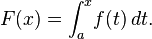The FTC states that F'(x) = f(x). That is, derivatives and integrals are inverse operations.

Unlike the previous theorems, the fundamental theorem of calculus provides a computational tool. It shows that you can solve integrals by constructing "antiderivatives."

### The fundamental theorem of linear algebra

Not everyone knows about the fundamental theorem of linear algebra, but there is an excellent 1993 article by Gil Strang that describes its importance. For an m x n matrix A, the theorem relates the dimensions of the row space of A (R(A)) and the nullspace of A (N(A)). The result is that dim(R(A)) + dim(N(A)) = n.

The theorem also describes four important subspaces and describes the geometry of A and At when thought of as linear transformations. The theorem shows that some subspaces are orthogonal to others. (Strang actually combines four theorems into his statement of the Fundamental Theorem, including a theorem that motivates the statistical practice of ordinary least squares.)

### The fundamental theorem of statistics

Although most statistical textbooks do not single out a result as THE fundamental theorem of statistics, I can think of two results that could make a claim to the title. These results are based in probability theory, so perhaps they are more aptly named fundamental theorems of probability.

• The Law of Large Numbers (LLN) provides the mathematical basis for understanding random events. The LLN says that if you repeat a trial many times, then the average of the observed values tend to be close to the expected value. (In general, the more trials you run, the better the estimates.) For example, you toss a fair die many times and compute the average of the numbers that appear. The average should converge to 3.5, which is the expected value of the roll because (1+2+3+4+5+6)/6 = 3.5. The same theorem ensures that about one-sixth of the faces are 1s, one-sixth are 2s, and so forth.
• The Central Limit theorem (CLT) states that the mean of a sample of size n is approximately normally distributed when n is large. Perhaps more importantly, the CLT provides the mean and the standard deviation of the sampling distribution in terms of the sample size, the population mean μ, and the population variance σ2. Specifically, the sampling distribution of the mean is approximately normally distributed with mean μ and standard deviation σ/sqrt(n).

Of these, the Central Limit theorem gets my vote for being the Fundamental Theorem of Statistics. The LLN is important, but hardly surprising. It is the basis for frequentist statistics and assures us that large random samples tend to reflect the population. In contrast, the CLT is surprising because the sampling distribution of the mean is approximately normal regardless of the distribution of the original data! As a bonus, the CLT can be used computationally. It forms the basis for many statistical tests by estimating the accuracy of a statistical estimate. Lastly, the CLT connects important concepts in statistics: means, variances, sample size, and accuracy of point estimates.

Do you have a favorite "Fundamental Theorem"? Do you marvel at an applied theorem such as the fundamental theorem of linear programming or chuckle at a pseudo-theorems such as the fundamental theorem of software engineering? Share your thoughts in the comments.

tags: History, Just for Fun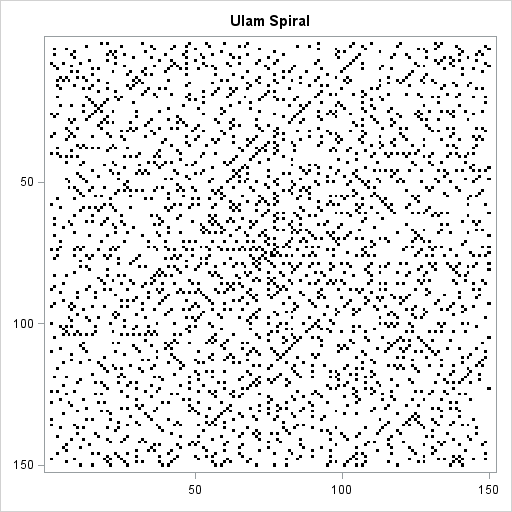Prime numbers are strange beasts. They exhibit properties of both randomness and regularity. Recently I watched an excellent nine-minute video on the Numberphile video blog that shows that if you write the natural numbers in a spiral pattern (called the Ulam spiral), then there are certain lines in the pattern that are very likely to contain prime numbers.

The image to the left shows the first 22,500 natural numbers arranged in the Ulam spiral pattern within a 150 x 150 grid. Cells that contain prime numbers are colored black. Cells that contain composite numbers are colored white. You can see certain diagonal lines with slope ±1 that contain a large number of prime numbers. There are conjectures in number theory that explain why certain lines along the Ulam spiral have a greater density of prime numbers than other lines. (The diagonal lines in the spiral correspond to quadratic equations.)

I don't know enough about prime numbers to blog about the mathematical properties of the Ulam spiral, but as soon as I saw the Ulam spiral explained, I knew that I wanted to generate it in SAS. The Ulam spiral packs the natural numbers into a square matrix in a certain order. This post describes how to construct the Ulam spiral in the SAS/IML matrix language.

### The Ulam spiral constructionThe Ulam spiral is simple to describe. On a gridded piece of paper, write down the number 1. Then write successive integers as you spiral out in a counter-clockwise fashion, as shown in the accompanying figure.

Although you can stop writing numbers at any time, for the purpose of this post let's assume you want to fill an N x N array with numbers. Notice that when N is even, the last number in the array (N2=4, 16, 36,...), appears in the upper left corner of the array, whereas for odd N, the last number (9, 25, 49,...) appears in the lower right corner. I found this asymmetry hard to deal with, so I created an algorithm that fills the N x N matrix so that the N2 term is always in the upper left. For odd values of N, the algorithm rotates the matrix after inserting the elements. I've previously shown that it is easy to write SAS/IML statements that rotate elements in a matrix.

My algorithm constructs the spiral iteratively. Notice that if you have constructed the spiral correctly for an (N–2) x (N–2) array, you can construct the N x N array by adding two vertical columns (first and last) and two horizontal rows (first and last). I call the outer rows and columns a "frame" because they remind me of a picture frame. Given a value for N, you can figure out the starting and ending values of each row and column of the frame. You can use the SAS/IML index creation operator to create each row and column, as shown in the following program:

```proc iml; start SpiralFrame(n); if n=1 then return(1); if n=2 then return({4 3, 1 2}); if n=3 then return({9 8 7, 2 1 6, 3 4 5}); X = j(n,n,.); /* top of frame. 's' means 'start'. 'e' means 'end' */ s = n##2; e = s - n + 2; X[1,1:n-1] = s:e; /* right side of frame */ s = e - 1; e = s - n + 2; X[1:n-1,n] = (s:e)`; /* bottom of frame */ s = e - 1; e = s - n + 2; X[n,n:2] = s:e; /* left side of frame */ s = e - 1; e = s - n + 2; X[n:2,1] = (s:e)`; return( X ); finish;   /* test the frame construction */ M2 = SpiralFrame(2); M4 = SpiralFrame(4); M6 = SpiralFrame(6); print M2, M4, M6;```You can see that the M4 matrix fits inside the M6 frame. Similarly, the M2 matrix fits inside the M4 frame.

After writing the code that generates the frames, the rest of the construction is easy. Start by creating the frame of size N. Decrease N by 2 and iteratively create a frame of size N, taking care to insert the (N–2) x (N–2) array into the interior of the existing N x N array. After the array is filled, rotate the array by 180 degrees if N is odd. The following SAS/IML statements implement this algorithm:

```start Rot180(m); return( m[nrow(m):1,ncol(m):1] ); finish;   /* Create N x N integer matrix with elements arranged in an Ulam spiral */ start UlamSpiral(n); X = SpiralFrame(n); /* create outermost frame */ k = 2; do i = n-2 to 2 by -2; /* iteratively create smaller frames */ r = k:n-k+1; /* rows (and cols) to insert smaller frame */ X[r, r] = SpiralFrame(i); /* insert smaller frame */ k = k+1; end; if mod(n,2)=1 then return(Rot180(X)); return(X); finish;   U10 = UlamSpiral(10); /* test program by creating 10 x 10 matrix */ print U10[f=3.];```You might not have much need to generate Ulam spirals in your work, but this exercise demonstrates several important principles of SAS/IML programming:

• It is often convenient to calculate the first and last element of an array and to use the color index operator (:) to generate the vector.
• Notice that I generated the largest frame first and inserted the smaller frames inside of it. That is more efficient than generating the smaller frames and then using concatenation to grow the matrix.
• In the same way, sometimes an algorithm is simpler or more efficient to implement if your DO loops run in reverse order.

The heat map at the beginning of this article is created by using the new HEATMAPDISC subroutine in SAS/IML 13.1. I will blog about heat maps in a future post. If you have SAS/IML 13.1 and want to play with Ulam spirals yourself, you can download the SAS code used in this blog post.

tags: 13.1, Just for Fun